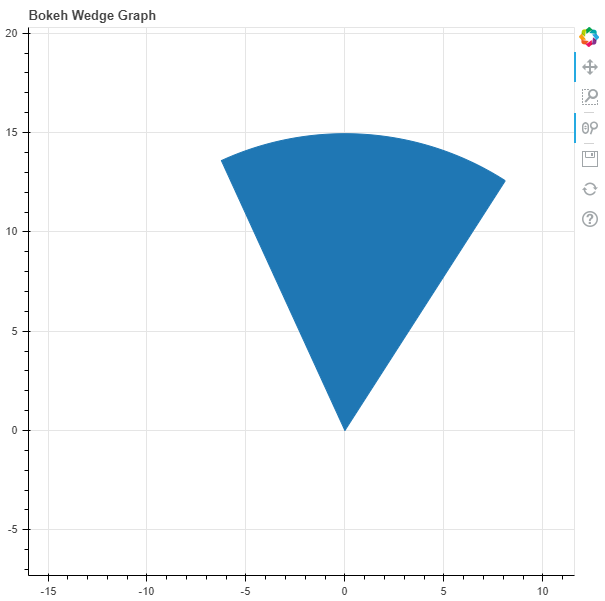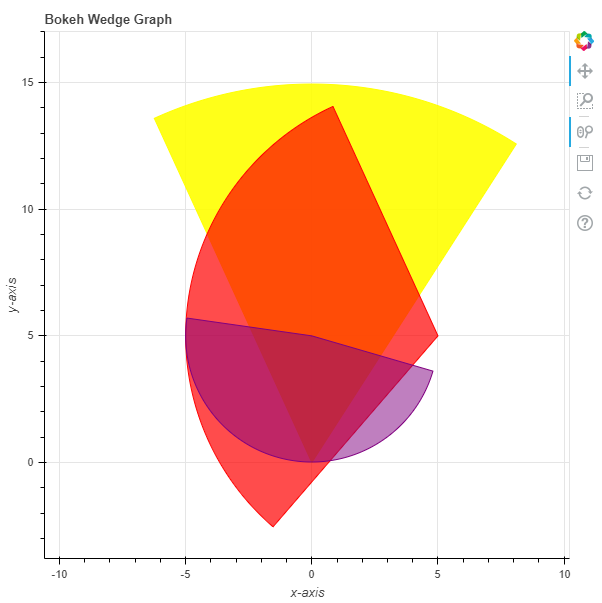# Python Bokeh – Plotting Wedges on a Graph

Bokeh is a Python interactive data visualization. It renders its plots using HTML and JavaScript. It targets modern web browsers for presentation providing elegant, concise construction of novel graphics with high-performance interactivity.

Bokeh can be used to plot wedges on a graph. Plotting wedges on a graph can be done using the `wedge()` method of the `plotting` module.

## plotting.figure.wedge()

Syntax : wedge(parameters)

Parameters :

• x : x-coordinates of the wedge markers
• y : y-coordinates of the wedge markers
• start_angle : angle to start the wedge markers
• start_angle_units : unit of the angle to start the wedge markers, default is radian
• end_angle : angle to end the wedge markers
• end_angle_units : unit of the angle to end the wedge markers
• direction : direction of strokes, default is anticlock

Returns : an object of class `GlyphRenderer`

Example 1 : In this example we will be using the default values for plotting the graph.

 `# importing the modules ` `from` `bokeh.plotting ``import` `figure, output_file, show ` `        `  `# file to save the model ` `output_file(``"gfg.html"``) ` `        `  `# instantiating the figure object ` `graph ``=` `figure(title ``=` `"Bokeh Wedge Graph"``) ` `      `  `# the points to be plotted ` `x ``=` `0` `y ``=` `0` ` `  `# radius of the wedge ` `radius ``=` `15` ` `  `# start angle of the wedge ` `start_angle ``=` `1` ` `  `# end angle of the wedge ` `end_angle ``=` `2` ` `  `# plotting the graph ` `graph.wedge(x, y, ` `            ``radius ``=` `radius, ` `            ``start_angle ``=` `start_angle, ` `            ``end_angle ``=` `end_angle) ` `      `  `# displaying the model ` `show(graph) `

Output :Example 2 : In this example we will be plotting multiple wedges with various other parameters.

 `# importing the modules ` `from` `bokeh.plotting ``import` `figure, output_file, show ` `        `  `# file to save the model ` `output_file(``"gfg.html"``) ` `        `  `# instantiating the figure object ` `graph ``=` `figure(title ``=` `"Bokeh Wedge Graph"``) ` ` `  `# name of the x-axis  ` `graph.xaxis.axis_label ``=` `"x-axis"` `       `  `# name of the y-axis  ` `graph.yaxis.axis_label ``=` `"y-axis"` ` `  `# the points to be plotted ` `x ``=` `[``0``, ``5``, ``0``] ` `y ``=` `[``0``, ``5``, ``5``] ` ` `  `# radius of the wedges ` `radius ``=` `[``15``, ``10``, ``5``] ` ` `  `# start angle of the wedges ` `start_angle ``=` `[``1``, ``2``, ``3``] ` ` `  `# end angle of the wedges ` `end_angle ``=` `[``2``, ``4``, ``6``] ` ` `  `# color value of the wedges ` `color ``=` `[``"yellow"``, ``"red"``, ``"purple"``] ` `  `  `# fill alpha value of the wedges ` `fill_alpha ``=` `[``0.9``, ``0.7``, ``0.5``] ` ` `  `# plotting the graph ` `graph.wedge(x, y, ` `            ``radius ``=` `radius, ` `            ``start_angle ``=` `start_angle, ` `            ``end_angle ``=` `end_angle, ` `            ``color ``=` `color, ` `            ``fill_alpha ``=` `fill_alpha) ` `      `  `# displaying the model ` `show(graph) `

Output :My Personal Notes arrow_drop_upCheck out this Author's contributed articles.

If you like GeeksforGeeks and would like to contribute, you can also write an article using contribute.geeksforgeeks.org or mail your article to contribute@geeksforgeeks.org. See your article appearing on the GeeksforGeeks main page and help other Geeks.

Please Improve this article if you find anything incorrect by clicking on the "Improve Article" button below.

Article Tags :

Be the First to upvote.

Please write to us at contribute@geeksforgeeks.org to report any issue with the above content.